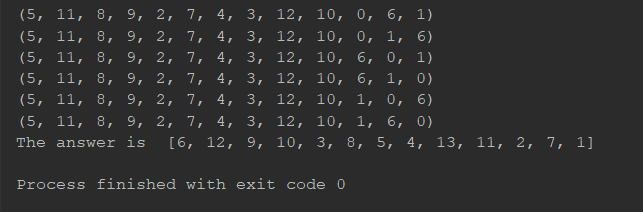Python 解置换群问题

Note: 作用两次的意思就是，当一个置换规则作用于字符串一次时，会生成一个新字符串。将这个规则作用在这个新字符串上，又会生成一个字符串，这个字符串就是两次作用的结果。

近世代数基础

$$\sigma = \left\{\begin{matrix}1 & 2 & 3 & 4 & 5 & 6 \\ 3 & 5 & 2 & 6 & 4 & 1\end{matrix}\right\}$$

$$\tau = \left\{\begin{matrix}1 & 3 & 4 \\ 3 & 4 & 1 \end{matrix}\right\}$$

$$\upsilon = \left\{\begin{matrix}1 & 2 & 3 & 4 & 5 & 6 \\ 6 & 4 & 2 & 3 & 5 & 1\end{matrix}\right\} = (1 6)(2 4 3)(5) = (1 6)(2 4 3) ​$$

(参考资料: 置换群题目汇总《近世代数》杨子胥)

暴力解法

import numpy as np
import itertools

# a is something like index
# b is the second output you want
# C (capital) is the first output we guess
a = np.array([1, 2, 3, 4, 5, 6, 7, 8, 9, 10, 11, 12, 13])
a = a-1
b = np.array([8, 7, 13, 11, 9, 4, 3, 10, 1, 2, 12, 5, 6])
b = b-1
c = np.zeros([a.size])

# traverse all permutations of a
for C in itertools.permutations(a, a.size):
print(C)
for num in C:
# c (lower case) is the second output in this iteration
c[num] = C[C[num]]
if (b == c).all():
C = [x+1 for x in C]
breakNote1: 这段代码中，我觉得我写得最棒的一句是c[num] = C[C[num]]。之前为了实现同样的功能，至少写了十几行代码，都是血泪……后来拜读了置换群题目汇总一文，才受到启发写成这个表达形式。

Note2: 如果原问题改成已知第三次输出，求第一次输出也很容易。只要加多一层中括号就可以了c[num] = C[C[C[num]]]。哈哈哈，运气好的话你的电脑可能要跑两个星期。(๑╹っ╹๑)

手算解法